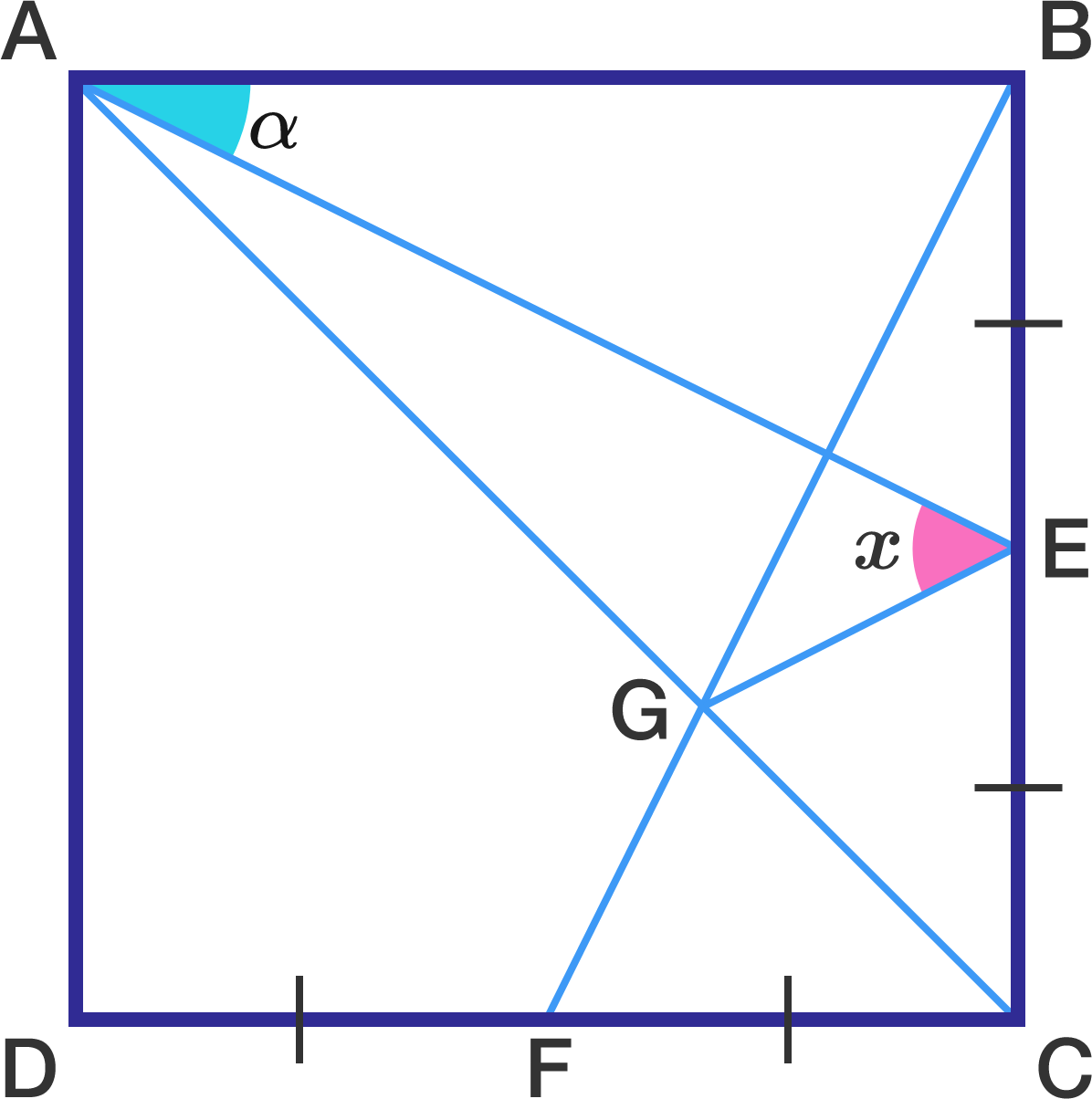Morning Square

Geometry Level 1In the square above, $E$ and $F$ are the midpoints of $\overline{BC}$ and $\overline{CD} ,$ respectively. If $G$ is the intersection point of $\overline{AC}$ and $\overline{BF}$ and $x=\angle AEG,$ find $x$ (in degrees) in terms of $\alpha.$

×